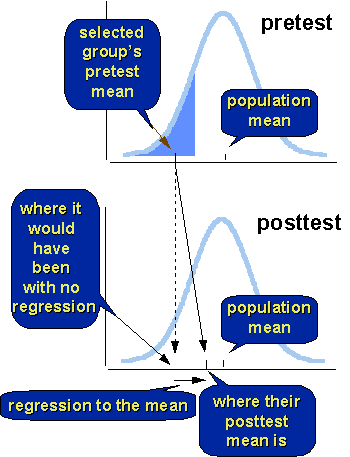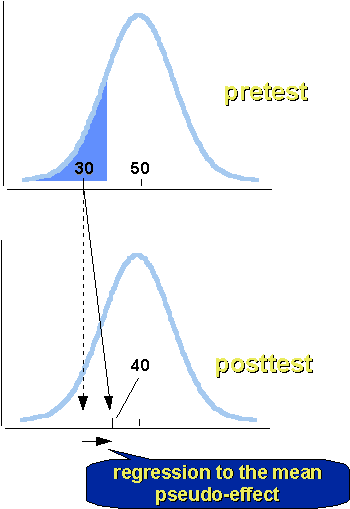Regression to the Mean

# Regression to the Mean

A regression threat, also known as a “regression artifact” or “regression to the mean” is a statistical phenomenon that occurs whenever you have a nonrandom sample from a population and two measures that are imperfectly correlated. The figure shows the regression to the mean phenomenon. The top part of the figure shows the pretest distribution for a population. Pretest scores are “normally” distributed, the frequency distribution looks like a “bell-shaped” curve. Assume that the sample for your study was selected exclusively from the low pretest scorers. You can see on the top part of the figure where their pretest mean is – clearly, it is considerably below the population average. What would we predict the posttest to look like? First, let’s assume that your program or treatment doesn’t work at all (the “null” case). Our naive assumption would be that our sample would score just as badly on the posttest as they did on the pretest. But they don’t! The bottom of the figure shows where the sample’s posttest mean would have been without regression and where it actually is. In actuality, the sample’s posttest mean wound up closer to the posttest population mean than their pretest mean was to the pretest population mean. In other words, the sample’s mean appears to regress toward the mean of the population from pretest to posttest.## Why Does It Happen?

Let’s start with a simple explanation and work from there. To see why regression to the mean happens, consider a concrete case. In your study you select the lowest 10% of the population based on their pretest score. What are the chances that on the posttest that exact group will once again constitute the lowest ten percent? Not likely. Most of them will probably be in the lowest ten percent on the posttest, but if even just a few are not, then their group’s mean will have to be closer to the population’s posttest than it was to the pretest. The same thing is true on the other end. If you select as your sample the highest ten percent pretest scorers, they aren’t likely to be the highest ten percent on the posttest (even though most of them may be in the top ten percent). If even just a few score below the top ten percent on the posttest their group’s posttest mean will have to be closer to the population posttest mean than to their pretest mean.

Here are a few things you need to know about the regression to the mean phenomenon:

• It is a statistical phenomenon.

Regression toward the mean occurs for two reasons. First, it results because you asymmetrically sampled from the population. If you randomly sample from the population, you would observe (subject to random error) that the population and your sample have the same pretest average. Because the sample is already at the population mean on the pretest, it is impossible for them to regress towards the mean of the population any more!

• It is a group phenomenon.

You cannot tell which way an individual’s score will move based on the regression to the mean phenomenon. Even though the group’s average will move toward the population’s, some individuals in the group are likely to move in the other direction.

• It happens between any two variables.

What you have to realize, is that the pattern of results I just described will happen anytime you measure two measures! It will happen forwards in time (i.e., from pretest to posttest). It will happen backwards in time (i.e., from posttest to pretest)! It will happen across measures collected at the same time (e.g., height and weight)! It will happen even if you don’t give your program or treatment.

• It is a relative phenomenon.

It has nothing to do with overall maturational trends. Notice in the figure above that I didn’t bother labeling the x-axis in either the pretest or posttest distribution. It could be that everyone in the population gains 20 points (on average) between the pretest and the posttest. But regression to the mean would still be operating, even in that case. That is, the low scorers would, on average, be gaining more than the population gain of 20 points (and thus their mean would be closer to the population’s).

• You can have regression up or down.

If your sample consists of below-population-mean scorers, the regression to the mean will make it appear that they move up on the other measure. But if your sample consists of high scorers, their mean will appear to move down relative to the population. (Note that even if their mean increases, they could be losing ground to the population. So, if a high-pretest-scoring sample gains five points on the posttest while the overall sample gains 15, we would suspect regression to the mean as an alternative explanation [to our program] for that relatively low change).

• The more extreme the sample group, the greater the regression to the mean.

If your sample differs from the population by only a little bit on the first measure, there won’t be much regression to the mean because there isn’t much room for them to regress – they’re already near the population mean. So, if you have a sample, even a nonrandom one, that is a pretty good subsample of the population, regression to the mean will be inconsequential (although it will be present). But if your sample is very extreme relative to the population (e.g., the lowest or highest x%), their mean is further from the population’s and has more room to regress.

• The less correlated the two variables, the greater the regression to the mean.

The other major factor that affects the amount of regression to the mean is the correlation between the two variables. If the two variables are perfectly correlated – the highest scorer on one is the highest on the other, next highest on one is next highest on the other, and so on – there will no be regression to the mean. But this is unlikely to ever occur in practice. We know from measurement theory that there is no such thing as “perfect” measurement – all measurement is assumed (under the true score model) to have some random error in measurement. It is only when the measure has no random error – is perfectly reliable – that we can expect it will be able to correlate perfectly. Since that just doesn’t happen in the real world, we have to assume that measures have some degree of unreliability, and that relationships between measures will not be perfect, and that there will appear to be regression to the mean between these two measures, given asymmetrically sampled subgroups.

## The Formula for the Percent of Regression to the Mean

You can estimate exactly the percent of regression to the mean in any given situation. The formula is:

$$Prm = 100(1 - r)$$

where:

• Prm = the percent of regression to the mean
• r = the correlation between the two measures

Consider the following four cases:

• if r = 1, there is no (i.e., 0%) regression to the mean
• if r = .5, there is 50% regression to the mean
• if r = .2, there is 80% regression to the mean
• if r = 0, there is 100% regression to the mean

In the first case, the two variables are perfectly correlated and there is no regression to the mean. With a correlation of .5, the sampled group moves fifty percent of the distance from the no-regression point to the mean of the population. If the correlation is a small .20, the sample will regress 80% of the distance. And, if there is no correlation between the measures, the sample will “regress” all the way back to the population mean! It’s worth thinking about what this last case means. With zero correlation, knowing a score on one measure gives you absolutely no information about the likely score for that person on the other measure. In that case, your best guess for how any person would perform on the second measure will be the mean of that second measure.

## Estimating and Correcting Regression to the Mean

Given our percentage formula, for any given situation we can estimate the regression to the mean. All we need to know is the mean of the sample on the first measure the population mean on both measures, and the correlation between measures. Consider a simple example. Here, we’ll assume that the pretest population mean is 50 and that we select a low-pretest scoring sample that has a mean of 30. To begin with, let’s assume that we do not give any program or treatment (i.e., the null case) and that the population is not changing over time on the characteristic being measured (i.e., steady-state). Given this, we would predict that the population mean would be 50 and that the sample would get a posttest score of 30 if there was no regression to the mean. Now, assume that the correlation is .50 between the pretest and posttest for the population. Given our formula, we would expect that the sampled group would regress 50% of the distance from the no-regression point to the population mean, or 50% of the way from 30 to 50. In this case, we would observe a score of 40 for the sampled group, which would constitute a 10-point pseudo-effect or regression artifact.Now, let’s relax some of the initial assumptions. For instance, let’s assume that between the pretest and posttest the population gained 15 points on average (and that this gain was uniform across the entire distribution, that is, the variance of the population stays the same across the two measurement occasions). In this case, a sample that had a pretest mean of 30 would be expected to get a posttest mean of 45 (i.e., 30+15) if there is no regression to the mean (i.e., r=1). But here, the correlation between pretest and posttest is .5 so we expect to see regression to the mean that covers 50% of the distance from the mean of 45 to the population posttest mean of 65. That is, we would observe a posttest average of 55 for our sample, again a pseudo-effect of 10 points.Regression to the mean is one of the trickiest threats to validity. It is subtle in its effects, and even excellent researchers sometimes fail to catch a potential regression artifact. You might want to learn more about the regression to the mean phenomenon. One good way to do that would be to simulate the phenomenon. If you’re not familiar with simulation, you can get a good introduction in the The Simulation Book. If you already understand the basic idea of simulation, you can do a manual (dice rolling) simulation of regression artifacts or a computerized simulation of regression artifacts.